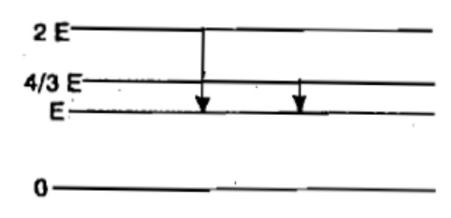The Bohr model of atoms 

(1) assumes that the angular momentum of electrons is quantised

(2) uses Einstein's photoelectric equation

(3) Predicts continuous emission spectra for atoms

(4) Predicts the same emission spectra for all types of atoms

Concept Questions :-

Bohr's model of atom
High Yielding Test Series + Question Bank - NEET 2020

Difficulty Level:

When a hydrogen atom is raised from the ground state to excited state

(1) both KE and PE increase

(2) both KE and PE decrease

(3) PE increases and KE decreases

(4) PE decreases and KE increases

Concept Questions :-

Bohr's model of atom
High Yielding Test Series + Question Bank - NEET 2020

Difficulty Level:

The extreme wavelengths of Paschen series are

(1) 0.365 $\mu m$ and 0.565 $\mu m$

(2) 0.818 $\mu m$ and 0.189 $\mu m$

(3) 1.45 $\mu m$ and 4.04 $\mu m$

(4) 2.27 $\mu m$ and 7.43 $\mu m$

Concept Questions :-

Spectral series
High Yielding Test Series + Question Bank - NEET 2020

Difficulty Level:

A hydrogen atom is in an excited state of principal quantum number (n), it emits a photon of wavelength ($\lambda$) when it returns to the ground state. The value of n is

(1) $\sqrt{\frac{\lambda R}{\lambda R-1}}$                   (2) $\sqrt{\frac{\left(\lambda R-1\right)}{\lambda R}}$

(3) $\sqrt{\lambda \left(R-1\right)}$                   (4) None of these

Concept Questions :-

Bohr's model of atom
High Yielding Test Series + Question Bank - NEET 2020

Difficulty Level:

Consider an electron in the nth orbit of a hydrogen atom in the Bohr model. The circumference of the orbit can be expressed in terms of the de-Broglie wavelength $\lambda$ of that electron as:

(1) (0.529) n$\lambda$           (2) $\sqrt{n\lambda }$             (3) (13.6) $\lambda$         (4) n$\lambda$

Concept Questions :-

Bohr's model of atom
High Yielding Test Series + Question Bank - NEET 2020

Difficulty Level:

In the Bohr's model of a hydrogen atom, the centripetal force is furnished by the Coulomb attraction between the proton and the electron.  If ${\alpha }_{0}$ is the radius of the ground state orbit, m is the mass and e is the charge on the electron, ${\epsilon }_{0}$ is the vaccum permittivity, the speed of the electron is  

(1) zero          (2) $\frac{e}{\sqrt{{\epsilon }_{0}{a}_{0}m}}$           (3) $\frac{e}{\sqrt{4{\mathrm{\pi \epsilon }}_{0}{\mathrm{a}}_{0}\mathrm{m}}}$               (4) $\frac{\sqrt{4{\mathrm{\pi \epsilon }}_{0}{\mathrm{a}}_{0}\mathrm{m}}}{e}$

Concept Questions :-

Bohr's model of atom
High Yielding Test Series + Question Bank - NEET 2020

Difficulty Level:

The ground state energy of H-atom is 13.6 eV. The energy needed to ionise H-atom from its second excited state 

(1) 1.51 eV          (2) 3.4 eV            (3) 13.6 eV             (4) 12.1 eV

Concept Questions :-

Bohr's model of atom
High Yielding Test Series + Question Bank - NEET 2020

Difficulty Level:

The element which has a ${k}_{a}$ x-rays line of wavelength 1.8 $\stackrel{0}{A}$ is

(1) Co, Z=27      (2) Iron, Z = 26        (3) Mn, Z = 25           (4) Ni, Z = 28

Concept Questions :-

Spectral series
High Yielding Test Series + Question Bank - NEET 2020

Difficulty Level:

The given diagram indicates the energy levels of a certain atom. When the system moves from 2E level to E, a photon of wavelength $\lambda$ is emitted. The wavelength of photon produced during its transition from $\frac{4E}{3}$ level to E is(1) $\frac{\lambda }{3}$           (2) $\frac{3\lambda }{4}$        (3) $\frac{4\lambda }{3}$            (4) $3\lambda$

Concept Questions :-

Spectral series
High Yielding Test Series + Question Bank - NEET 2020

Difficulty Level:

The wavelength of the first spectral line in the Balmer series of hydrogen atom is 6561 $\stackrel{0}{A}$. The wavelength of the second spectral line in the Balmer series of singly ionized helium atom is [IIT-JEE 2011]

(1) 1215 $\stackrel{0}{A}$        (2) 1640 $\stackrel{0}{A}$            (3) 2430 $\stackrel{0}{A}$           (4) 4687 $\stackrel{0}{A}$

Concept Questions :-

Spectral series